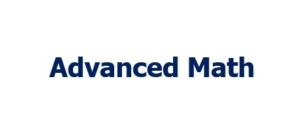2 week money back guarantee.### Why

Going deeper into Problem Solving allows students to develop a strategy mindset when they expand their thinking into multiple directions.

Math problems that students get in school are designed to teach concepts with repetition of many similar problems. Even if (as an example) a 6th grader takes up High School Math 1 (in school), they are still being taught concepts using problems that are not inherently challenging, they just learn concepts of higher Math levels but with very simple problems.

Our Advanced Math curriculum helps students expand on application of concepts into richer problem solving domains, requiring them to think differently about a solution.

With deeper problem solving aptitude, our Advanced Math students can learn any level Math (and other subjects) in a much shorter amount of time. They are able to achieve this because their mind has developed the strategic thinking needed to approach a concept from multiple directions. As a result they are able to take more number of rigorous courses (APs) in High School and excel without having to spend enormous amounts of time.

### Credentials

Our Advanced Math Students aced PSAT Math with a 99th percentile (most getting a full score), with zero dedicated preparation for PSAT.

Our Advanced Math 9th graders have aced the Math 1, Math 2, and Pre-Calculus CDM (Credit by Demonstrated Mastery), allowing them to skip those courses in High School and Middle School and advance.

All our Advanced Math students aced SAT with a Math Score of 780+ and a total score of 1500+.

### innovative approachA proprietary curriculum rich in problem solvingEngaging classrooms where students are encouraged to analyze problems from different perspectivesLow volume homework but with content rich in problem solving that challenges students' thinking without being repetitive.

Our curriculum will challenge your child beyond grade level math but in a very engaging and collaborative teaching style that draws on their problem solving skills without the high pressure of homework.

### Benefits your child may seeIncreased confidence in embracing new math concepts with a higher likelihood of success in problem solvingSince they will be mastering many concepts ahead of time, they will need to dedicate much less time to school math, in order to excel. The time saved they can apply to other subject areas for schoolThey will develop the good habits of persistence, detail orientation, creative thinking that will have positive impact in all walks of their student lives

### Starting Fall 2022

Adv MTH Level 1. Open Slot:

Spatial thinking problems, solving arithmetic problems within multiple constraints

Structured Problem Solving Strategies: Organized list, Guess and Check, Draw and Solve

Pre-Algebra & Simple Algebra equations

​Word problems involving arithmetic

​Shapes and Geometry

​Simple Venn Diagrams

Adv MTH Level 2. Thu 5:30PM:

​Grades: 3rd - 5th.

Structured Problem Solving Strategies: Organized Lists, Draw and Solve

Introduction to Algebra

Problems in Linear Algebra

Application of Venn Diagrams

2D geometry: Polygons & Complex Shapes. Areas and Perimeter

2D geometry: Analytical Geometry (shape counting strategies)

​Patterns and Math Case Studies

Structured Problem Solving Strategies: Working Backwards.

Adv MTH Level 3. Mon 5:30PM:

​Grades: 4th - 6th.

Fractions, Ratios, Proportions

Problems in Time, Work, Speed, Distance

Problems in Linear Algebra with multi-variables. System of Equations

Advanced 2D geometry: Area and Perimeter strategies, Circles

​Patterns and sequences

Introduction to Functions

Adv MTH Level 4. High School Math 1. Thu 6:40PM:

Grades: 6th - 8th.

Exponents and Expressions (review)

Functions: Linear, Exponential

Geometry: Coordinates and Mid points

System of Equations, Inequalities, Compound Inequalities

Statistics and Interpreting Data

Non Linear Functions (Quadratic and Polynomials)

Adv MTH Level 5A: High School Math 2. Wed 6:40PM

Grades: 7th - 10th.

Functions: Linear and Exponential Functions, Direct and Inverse Relations, Radicals and Radical Expressions

Quadratics: Solving Quadratics by Graphing, Factoring, Completing the Square, Quadratic Formula, Applications

Complex Numbers: Pure Imaginary Numbers, Powers of i, Operations with Complex Numbers

Complex Inequalities

Geometry: Transformations, Triangle Congruence, Triangle Similarity, Right Triangles Geometry

Statistics and Probability: Simple, Conditional

Introduction to Trigonometry

Adv MTH Level 5B: High School Math 3. Thu 5:30PM.

Grades: 7th - 10th.

Radical Expressions, Absolute Values, Complex Numbers (Review)

Functions and Polynomials: Features & Graphs, Parent Functions, Piecewise functions and applications, Inverse functions & graphs, Combining functions, Polynomial Division / Synthetic Division, Rate of Change, Remainder Theorem, Solving Polynomials, Simplifying Rational Expressions

Rational Expressions & Equations: Arithmetic Operations, Solving, Graphing

Logarithms: Fundamentals, Graphing, Applications

Geometry: Triangle Centers, Quadrilaterals, Surface Area, Volume , Density

Trigonometry: Unit Circle, Periodic data, Graphing, Translating functions, Applications

Circles: Tangents, Chords and Arcs, Inscribed Angles, Sector Areas, Circle Equations

Statistics & Data Analysis: Fundamentals (Mean, variance, Deviation), Normal distribution, Margin of Error, Sampling

Adv MTH Level 6: Pre-calculus. Open slots:

Conics (Parabola, Ellipse, Hyperbola)

Advanced Logarithms and Exponents

Advanced Trigonometry and applications

Vectors and Complex Number Algebra

Calculus: Limits

Adv MTH Level 7: AP Calculus AB/BC. Open Slots:

Limits & Continuity

Foundations of Differentiation & Differentiability

Complex Differentiation and Higher Order Derivatives

Applications of Differentiation

Differential Equations

Fundamentals of Integration

Applications of Integration (arc length, area and volume)

Infinite Series

Parametric and Polar expressions including arc length, area, volume.

Pricing (per 4 weeks)

Grades 1 - 8, High School Math 1, Math 2, Math 3: \$135

*Adv MTH Level 6 (Pre-Calculus)(90 mins a week): \$200

*Adv MTH Level 7 (AP Calculus AB+BC) (2 hours a week): \$250

Private Classes:
4weeks: \$300 for 1 hr a week and \$600 for 2 hrs a week.

See registration page for applicable discounts

### Schedule a FREE assessment

Thinking Feet’s main learning center is located in the Ballantyne area in Mecklenburg county. We are just minutes away from nearby Blakeney, Weddington, Marvin, Waxhaw, Pineville, Matthews, Wesley Chapel, Piper Glen and Fort Mill, SC.​​​​